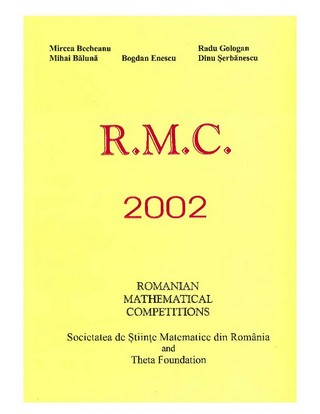### Book Toankho

Sách hay - Sách đẹp

Browsing:

## Romanian Mathematical Competitions 2007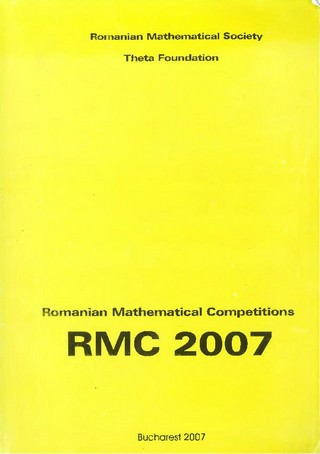## USA and International Mathematical Olympiads 2004The Mathematical Olympiad examinations, covering the USA Mathematical Olympiad (USAMO) and the International Mathematical Olypiad (IMO), have been published annually since 1976. The IMO is the world mathematics championship for high school students. It takes place every year in a Read more…

## 103 Trigonometry Problems (From the Training of the USA IMO Team)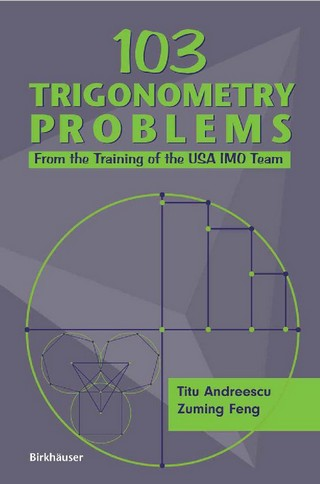* Problem-solving tactics and practical test-taking techniques provide in-depth enrichment and preparation for various math competitions * Comprehensive introduction to trigonometric functions, their relations and functional properties, and their applications in the Euclidean plane and solid geometry * A cogent Read more…

## Romanian Mathematical Competitions 1998## Lecture Notes on Mathematical Olympiad Courses (For Junior Section, Vol. 2)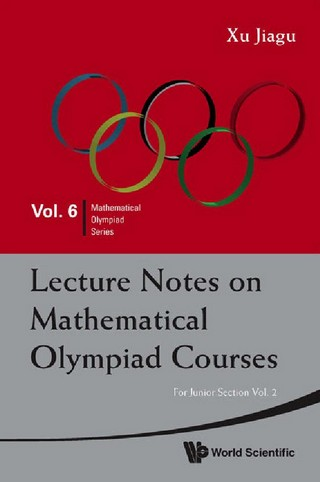Olympiad mathematics is not a collection of techniques of solving mathematical problems but a system for advancing mathematical education. This book is based on the lecture notes of the mathematical Olympiad training courses conducted by the author in Singapore. Its Read more…

## Mathematical Diamonds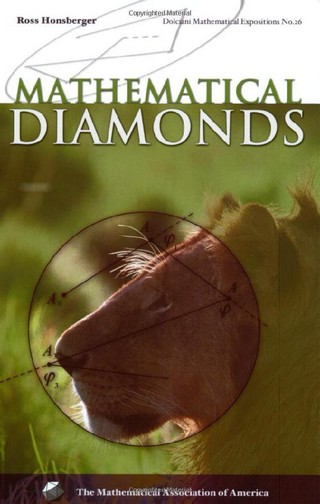Ross Honsberger has done it again. He has brought together another wonderful collection of elementary mathematical problems and their solutions abounding in striking surprises and brilliant ideas that reflect the beauty of mathematics. Many of these problems come from mathematical Read more…

## Lecture Notes on Mathematical Olympiad Courses (For Junior Section, Vol. 1)Olympiad mathematics is not a collection of techniques of solving mathematical problems but a system for advancing mathematical education. This book is based on the lecture notes of the mathematical Olympiad training courses conducted by the author in Singapore. Its Read more…

## Romanian Mathematical Competitions 2002